Note: This is an archvied version of our old webpage. Some links might be broken. The current one can be found here.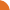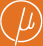Chair for Foundations of Software Reliability and Theoretical Computer Science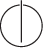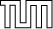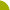Publications - On Fixed Point Equations over Commutative SemiringsReference:

Javier Esparza, Stefan Kiefer, and Michael Luttenberger. On fixed point equations over commutative semirings. In Wolfgang Thomas and Pascal Weil, editors, Proceedings of the 24th International Symposium on Theoretical Aspects of Computer Science (STACS), volume 4393 of LNCS, pages 296–307, Aachen, Germany, 2007. Springer.

Abstract:

Fixed point equations x = f(x) over omega-continuous semirings can be seen as the mathematical foundation of interprocedural program analysis. The sequence 0, f(0), f(f(0)), ... converges to the least fixed point mu.f. The convergence can be accelerated if the underlying semiring is commutative. We show that accelerations in the literature, namely Newton's method for the arithmetic semiring and an acceleration for commutative Kleene algebras due to Hopkins and Kozen, are instances of a general algorithm for arbitrary commutative omega-continuous semirings. In a second contribution, we improve the O(3^n) bound of Hopkins and Kozen and show that their acceleration reaches mu.f after n iterations, where n is the number of equations. Finally, we apply the Hopkins-Kozen acceleration to itself and study the resulting hierarchy of increasingly fast accelerations.

Suggested BibTeX entry:

@inproceedings{EKL07,
author = {Javier Esparza and Stefan Kiefer and Michael Luttenberger},
booktitle = {Proceedings of the 24th International Symposium on Theoretical Aspects of Computer Science (STACS)},
editor = {Wolfgang Thomas and Pascal Weil},
pages = {296--307},
publisher = {Springer},
series = {LNCS},
title = {On Fixed Point Equations over Commutative Semirings},
volume = {4393},
year = {2007}
}GZipped PostScript (179 kB)PDF (162 kB)Tech report version